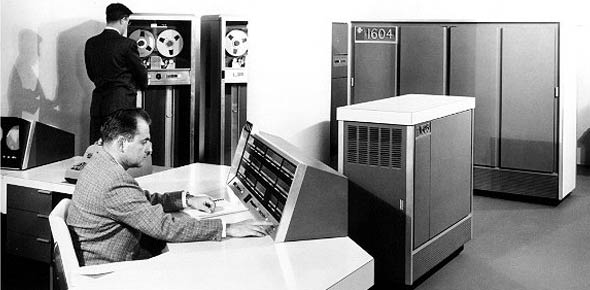# CDC Volume 2 UREs 2AX5X

100 Questions | Total Attempts: 244Settings• 1.
Atmospheric pressure at sea level is?
• A.

14.7 PSI

• B.

17.4 PSI

• C.

29.92 PSI

• D.

29.29 PSI

• 2.
A barometer is designed to measure pressure in?
• A.

Pounds per square inch

• B.

Inches of mercury

• C.

Milibars of alcohol

• D.

Ounces of air

• 3.
The primary force an airfoil has to defeat is
• A.

Lift

• B.

Drag

• C.

Thrust

• D.

Weight

• 4.
The force that acts upward at a right angle to the direction of the relative wind is
• A.

Lift

• B.

Drag

• C.

Thrust

• D.

Weight

• 5.
The force that acts parallel to the relative wind is
• A.

Lift

• B.

Drag

• C.

Thrust

• D.

Weight

• 6.
The stress that resists the force tending to cause one layer of material to slide over an adjacent layer is called
• A.

Bending

• B.

Tension

• C.

Torsion

• D.

Shear

• 7.
What is the design that uses any combination of longerons, stringers, bulkheads, and frames in the construction of the fuselage called?
• A.

Fuselage

• B.

Longerons

• C.

Monocoque

• D.

Semi-monocoque

• 8.
The structural members used to give shape to the aircraft and provide a means for attaching the skin to the structure are called
• A.

Longerons

• B.

• C.

Stringers

• D.

Frames

• 9.
The most common type of material used for fuselage construction is
• A.

Steel

• B.

Titanium

• C.

Magnesium

• D.

Aluminum alloy

• 10.
The wing design used on most modern military aircraft is
• A.

Cantilever

• B.

Monocoque

• C.

External braced

• D.

Semi-monocoque

• 11.
The fixed vertical surface of the empennage is called the
• A.

Stabilator

• B.

Elevator

• C.

Rudder

• D.

Fin

• 12.
Engine mounts are typically made of corrosion-resistant steel that is able to withstand forces of
• A.

120,000 to 140,000 PSI

• B.

150,000 to 170,000 PSI

• C.

180,000 to 200,000 PSI

• D.

210,000 to 230,000 PSI

• 13.
The main difference between inspection plates and panels is
• A.

Size

• B.

Material

• C.

Location

• D.

Installation

• 14.
A network of ultrafine cracks that form on or under the surface of transparent plastics is called
• A.

Crazing

• B.

Casting

• C.

Fretting

• D.

Pitting

• 15.
What are the reference locations in the vertical direction measured from a reference datum that is usually well below the aircraft called?
• A.

Buttlines

• B.

Waterlines

• C.

Buttock stations

• D.

Fuselage stations

• 16.
Movement about the vertical axis is called
• A.

Roll

• B.

Yaw

• C.

Crab

• D.

Pitch

• 17.
Which flight control surface controls the aircraft about the longitudinal axis?
• A.

Rudder

• B.

Aileron

• C.

Elevator

• D.

Wing flaps

• 18.
Which of these is attached to the trailing edge of a horizontal stabilizer?
• A.

Flap

• B.

Spoiler

• C.

Rudder

• D.

Elevator

• 19.
When making a right turn, the relative position of the ailerons id the right aileron trailing edge is
• A.

Up, left aileron trailing edge is up

• B.

Up, left aileron trailing edge is down

• C.

Down, left aileron trailing edge is up

• D.

Down, left aileron trailing edge is down

• 20.
The purpose of wing flaps is to
• A.

Increase lift and drag

• B.

Decrease lift and drag

• C.

Increase lift and decrease drag

• D.

Decrease lift and increase drag

• 21.
The purpose of aircraft trim system is to
• A.

Reduce the amount of lift

• B.

Prevent the aircraft from stalling

• C.

Correct minor deviations in flight attitude

• D.

Improve augmented lateral control surface movement

• 22.
Matter is any substance that has mass and
• A.

Can be seen

• B.

Occupies space

• C.

Has constant volume

• D.

Cannot be compressed

• 23.
• A.

Molecules, protons, neutrons

• B.

Protons, molecules, electrons

• C.

Molecules, neutrons, electrons

• D.

Protons, neutrons, electrons

• 24.
Electrons can be released from their order orbit by all of the following forces except
• A.

Heat

• B.

Cold

• C.

Chemical action

• D.

Changing magnetic field

• 25.
What is the electrical pressure that causes electrons to flow through the conductor called?
• A.

Chemical swap

• B.

Crossfeed field

• C.

Electromotive force

• D.

Transferring magnetic field

Related TopicsBack to top